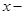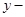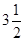# CLASS 8 MATHS CHAPTER-15 INTRODUCTION TO GRAPHS

### Exercise 15.1

Question 1.

The following graph shows the temperature of a patient in a hospital, recorded every hour:

(a) What was the patient’s temperature at 1 p.m.?

(b) When was the patient’s temperature 38.5° C?

(c) The patient’s temperature was the same two times during the period given. What were these two times?

(d) What was the temperature at 1.30 p.m.? How did you arrive at your answer?

(e) During which periods did the patients’ temperature showed an upward trend?

solution :

(a) The patient’s temperature was 36.5C at 1 p.m.

(b) The patient’s temperature was 38.5C at 12 noon.

(c) The patient’s temperature was same at 1 p.m. and 2 p.m.

(d) The temperature at 1.30 p.m. is 36.5C. The point between 1 p.m. and 2 p.m.,axis is equidistant from the two points showing 1 p.m. and 2 p.m. So it represents 1.30 p.m. Similarly the point onaxis, between 36o C and 37C will represent 36.5o C.

(e) The patient’s temperature showed an upward trend from 9 a.m. to 11 a.m.

Question 2.
The following line graph shows the yearly sales figures for a manufacturing company.

(a) What were the sales in (i) 2002 (ii) 2006?

(b) What were the sales in (i) 2003 (ii) 2005?

(c) Compute the difference between the sales in 2002 and 2006.

(d) In which year was there the greatest difference between the sales as compared
to its previous year?

solution :

(a) The sales in:

(i) 2002 was Rs.4 crores and (ii) 2006 was Rs.8crores.

(b) The sales in:

(i) 2003 was Rs.7crores

(ii) 2005 was Rs.10crores.

(c) The difference of sales in 2002 and 2006 = Rs.8crores – Rs.4crores = Rs.4crores

(d) In the year 2005, there was the greatest difference between the sales as compared to its previous year, which is (Rs.10crores – Rs.6crores) = Rs.4crores.

Question 3.

For an experiment in Botany, two different plants, plant A and plant B were grownunder similar laboratory conditions. Their heights were measured at the end of each week for 3 weeks. The results are shown by the following graph.

(a) How high was Plant A after (i) 2 weeks (ii) 3 weeks?

(b) How high was Plant B after (i) 2 weeks (ii) 3 weeks?

(c) How much did Plant A grow during the 3rd week?

(d) How much did Plant B grow from the end of the 2nd week to the end of the 3rd week?

(e) During which week did Plant A grow most?

(f) During which week did Plant B grow least?

(g) Were the two plants of the same height during any week shown here? Specify.

solution :

(a) (i) The plant A was 7 cm high after 2 weeks and

(ii) after 3 weeks it was 9 cm high.

(b) (i) Plant B was also 7 cm high after 2 weeks and

(ii) after 3 weeks it was 10 cm high.

(c) Plant A grew = 9 cm – 7 cm = 2 cm during 3rd week.

(d) Plant B grew during end of the 2nd week to the end of the 3rd week

= 10 cm – 7 cm = 3 cm.

(e) Plant A grew the highest during second week.

(f) Plant B grew the least during first week.

(g) At the end of the second week, plant A and B were of the same height.

Question 4.

The following graph shows the temperature forecast and the actual temperature for
each day of a week.

(a) On which days was the forecast temperature the same as the actual temperature?

(b) What was the maximum forecast temperature during the week?

(c) What was the minimum actual temperature during the week?

(d) On which day did the actual temperature differ the most from the forecast
temperature?

solution :

(a) On Tuesday, Friday and Sunday, the forecast temperature was same as the actual temperature.

(b) The maximum forecast temperature was 35o C.

(c) The minimum actual temperature was 15o C.

(d) The actual temperature differed the most from the forecast temperature on Thursday.

Question 5.
Use the tables below to draw linear graphs.

(a) The number of days a hill side city received snow in different years.

(b) Population (in thousands) of men and women in a village in different years.

solution :

(a)

(b)

Question 6.
A courier-person cycles from a town to a neighbouring suburban area to deliver a parcel to a merchant. His distance from the town at different times is shown by the following graph.

(a) What is the scale taken for the time axis?

(b) How much time did the person take for the travel?

(c) How far is the place of the merchant from the town?

(d) Did the person stop on his way? Explain.

(e) During which period did he ride fastest?

solution :

(a) 4 units = 1 hour.

(b) The person tookhours for the travel.

(c) It was 22 km far from the town.

(d) Yes, this has been indicated by the horizontal part of the graph. He stayed from 10 am to 10.30 am.

(e) He rode the fastest between 8 am and 9 am.

Question 7.

solution :

(i) It is showing the increase in temperature.

(ii) It is showing the decrease in temperature.

(iii) The graph figure (iii) is not possible since temperature is increasing very rapidly which is not possible.

(iv) It is showing constant temperature.

### NCERT Solutions for Class 8 Maths Exercise 15.2

Question 1.
Plot the following points on a graph sheet. Verify if they lie on a line

(a) A(4,0), B(4,2), C(4,6), D(4, 2.5)

(b) P(1,1), Q(2,2), R(3,3), S(4,4)

(c) K(2, 3), L(5, 3), M(5, 5), N(2, 5)

solution :

(a) All points A, B, C and D lie on a vertical line.

(b) P, Q, R and S points also make a line. It verifies that these points lie on a line.

(c) These points do not lie in a straight line.

Question 2.
Draw the line passing through (2, 3) and (3, 2). Find the coordinates of the points at which this line meets the x-axis and y-axis.

solution :

The coordinates of the points at which this line meets theaxis at (5, 0) andaxis at (0, 5).

Question 3.

Write the coordinates of the vertices of each of these adjoining figures.

solution :

Vertices of figure OABC

O (0, 0), A (2, 0), B (2, 3) and C (0, 3)

Vertices of figure PQRS

P (4, 3), Q (6, 1), R (6, 5) and S (4, 7)

Vertices of figure LMK

L (7, 7), M (10, 8) and K (10, 5)

Question 4.
State whether True or False. Correct that are false.

(i) A point whose x coordinate is zero and y-coordinate is non-zero will lie on the y-axis.

(ii) A point whose y coordinate is zero and x-coordinate is 5 will lie on y-axis.

(iii) The coordinates of the origin are (0, 0).

Ans. (i) True (ii) False, it will lie onaxis. (iii) True

### NCERT Solutions for Class 8 Maths Exercise 15.3

Question 1.
Draw the graphs for the following tables of values, with suitable scales on the axes.

(a) Cost of apples

(i) Does the graph pass through the origin?
(ii)Use the graph to find the interest on Rs 2500 for a year.
(iii)To get an interest of Rs 280 per year, how much money should be deposited?

solution :

(a)

(b) (i) The car covered 20 km distance.

(ii)It was 7.30 am, when it covered 100 km distance.

(c) (i) Yes, the graph passes through the origin.

(ii) Interest on `2500 is `200 for a year.

(iii) `3500 should be deposited for interest of `280.

Question 2.
Draw a graph for the following.

solution :
(i) Yes, it is a linear graph.

(ii) No, it is not a linear graph because the graph does not provide a straight line.

Get 30% off your first purchase!

X
error: Content is protected !!
Scroll to Top